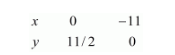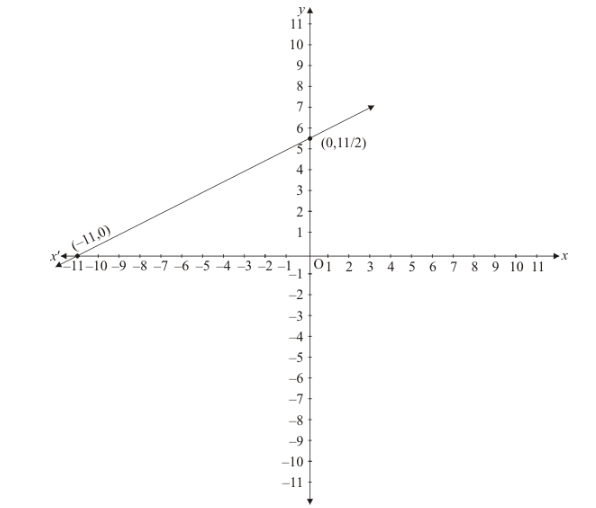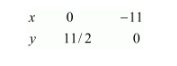# Show graphically that each one of the following systems of equations has infinitely many solutions:`
Question:

Show graphically that each one of the following systems of equations has infinitely many solutions:

$x-2 y+11=0$

$3 x-6 y+33=0$

Solution:

The given equations are

$x-2 y+11=0$ $\ldots \ldots \ldots(i)$

$3 x-6 y+33=0$(ii)

Putting $x=0$ in equation $(i)$, we get:

$\Rightarrow 0-2 y=-11$

$\Rightarrow y=11 / 2$

$x=0, \quad y=11 / 2$

Putting $y=0$ in equation $(i)$ we get:

$\Rightarrow x-2 \times=-11$

$\Rightarrow x=-11$

$x=-11, \quad y=0$

Use the following table to draw the graph.Draw the graph by plotting the two points $A(0,11 / 2), B(-11,0)$ from table.Graph of the equation….$(i i)$

$3 x-6 y=-33$....$(i i)$

Putting $x=0$ in equation (ii) we get:

$\Rightarrow 3 \times 0-6 y=-33$

$\Rightarrow y=11 / 2$

$x=0, \quad y=11 / 2$

Putting $y=0$ in equation $(i i)$, we get

$\Rightarrow 3 x-6 \times 0=-33$

$\Rightarrow x=-11$

$x=-11, \quad y=0$

Use the following table to draw the graph.Draw the graph by plotting the two points $C(0,11 / 2), D(-11,0)$ from table.

Thus the graph of the two equations are coincide

Consequently, every solution of one equation is a solution of the other.

Hence the equations have infinitely many solutions.